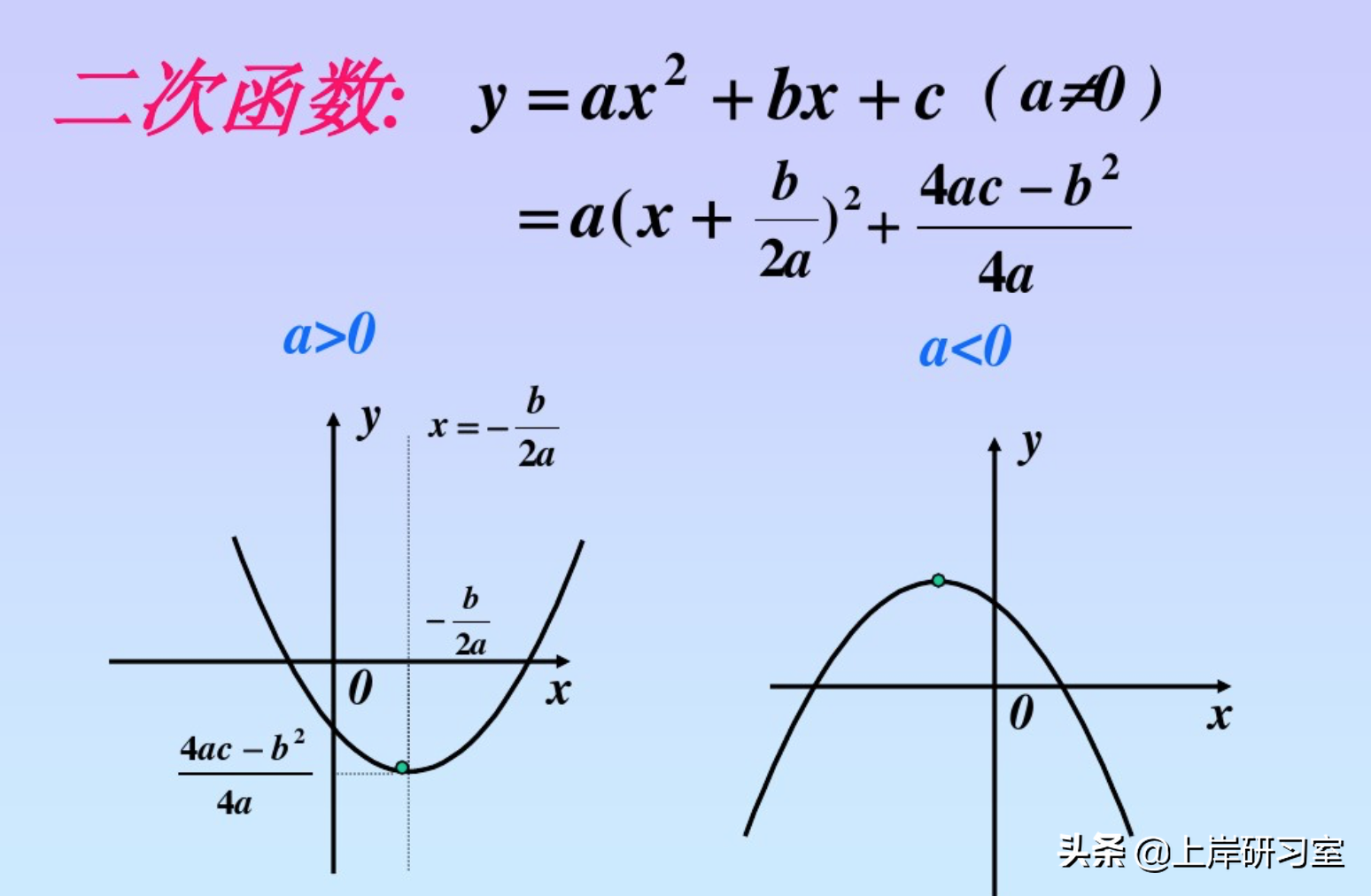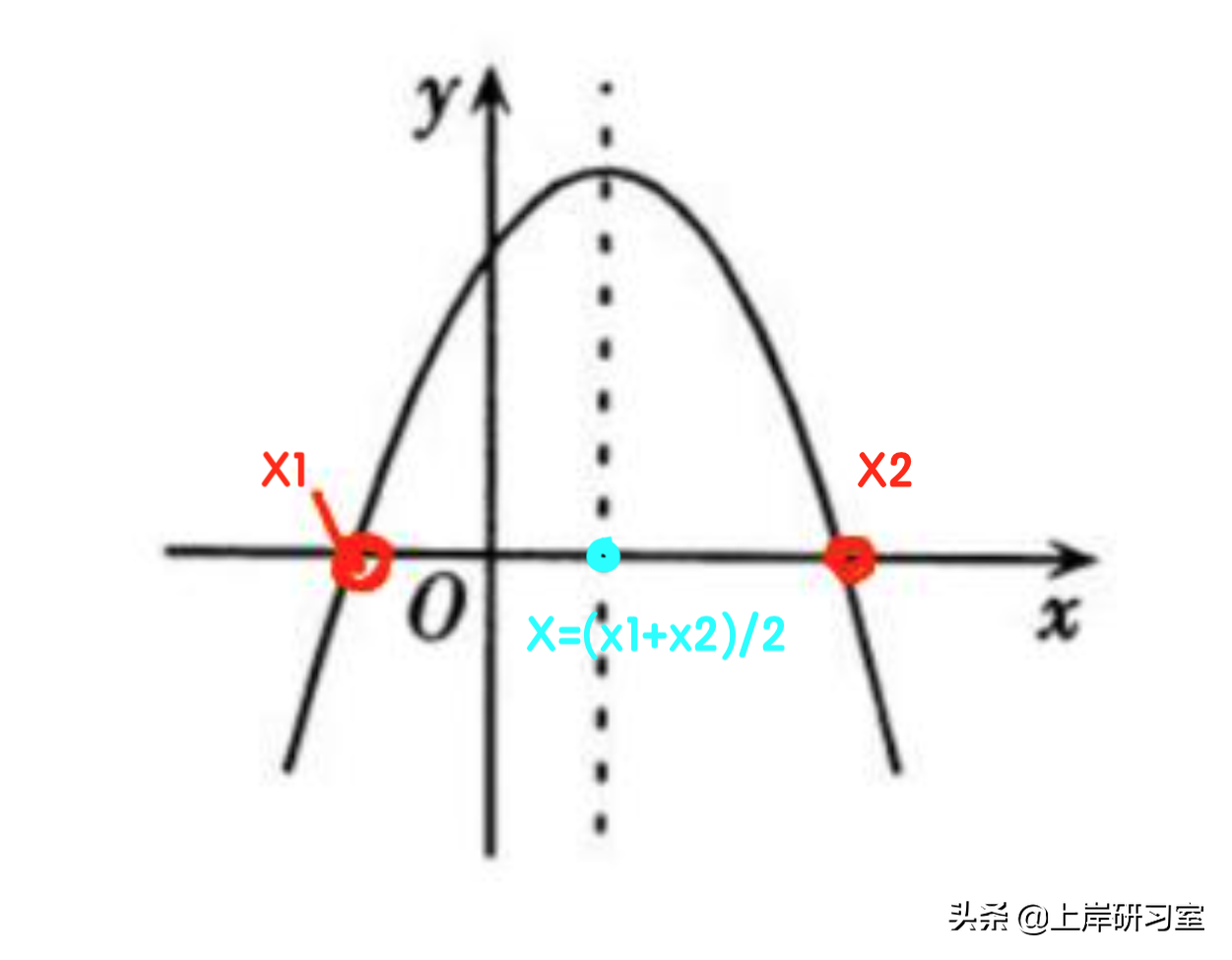# 二次函数最大值公式「建议收藏」

1.二次函数表达式为y=ax²+bx+c（且a≠0），它是一个二次多项式（或单项式）。

2.题型特征:常以经济利润的模型相结合的方式进行考察。

①直接给出函数表达式求最值。

A.100 B.150 C.200 D.250

(1)题目中直接给出了函数关系P=10000+400Q-Q2 ，整理可得P=-Q2+400Q+10000。

(2)直接运用结论：当 Q=-b/2a→(-400)/(-1*2)=200 时，取得最大值，因此选择C选项。②此消彼长，薄利多销型

1.根据条件和所求列方程，写成两括号相乘的形式。

2.求出使方程等于 0 时的两个根:x1、x2。

3.求出两个的平均值(对称轴):当 x=(x1+x2)/2 时，此时乘积最大。A.100 元 B.102 元 C.105 元 D.108 元

(1)根据总利润=单个商品利润 * 销量，商品成本为70，原单价为120，原单利为120-70=50。

(2)假设降价 x 次，即降价 x 元，利润为 50-x，销量为 100+5x，可列式 利润=(50-x)*(100+5x)。令 y=0，50-x=0→x1=50，100+5x=0→x2=-20，取中 x=(50-20)/2=15。

(3)x解得=15，即降价 15 次，共降 15 元，因此现单价=120-15=105 元。所以这道题选择C选项。

A.144 B.136 C.128 D.142

(1)根据题意成本为 144，售价为 200，利润为 200-144=56 元。

(2)假设降价 x 次，则降价 2x 元，销量增加 6x 套。利润 y=单利*销量=(56-2x)(120+6x)，令 y=0，56-2x=0→x1=56/2=28，120+6x=0→x2=-20，取中 x=(28-20)/2=4。

(3)降价 4 次，销量增加 6*4=24 套，总销量=120+24=144 套。因此这道题选择A选项。

※如果大家刷题多了之后，这道题根据倍数特性可以直接秒杀选项。